Foxtable(狐表)用户栏目专家坐堂 → 请教

共有713人关注过本帖树形打印复制链接

# 主题：请教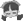1楼 | 信息 | 搜索 | 邮箱 | 主页 | UC加好友发短信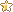2020年（农历庚子年）是中国传统的“鼠年”。 我们把只含数字2和0的自然数（包括0）称为“鼠数”，全体“鼠数” 自小至大排成的数列，称为“鼠数列”： 0，2，20，22，200，202，220，222，2000，? 一、第2020个“鼠数”是什么数？ 二、前2020个“鼠数”之和等于多少？ 三、前2020个“鼠数”中去掉开头的0，剩下的数之积的末尾一共有多少个0？ 四、前2020个“鼠数”中去掉开头的0，剩下的数从左至右写成一个数 A=220222002022202222000?。 那么，A是多少位数？ 五、A除以2020的余数是多少？能不能用程序生成这样的数组？我用EXCELS想了二天，只想到它们的部份数据关系是：=IF(ROW()<=673,2*(1-10^(ROW()-1))/(1-10),"")生成的数组是：2、22、222、2222、22222、2222222*10^(ROW()-1)*10+2*10^(ROW()-(COLUMN()-(COLUMN(\$A\$2)-1)))+2生成有数组是：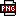此主题相关图片如下：qq图片20200205133307.png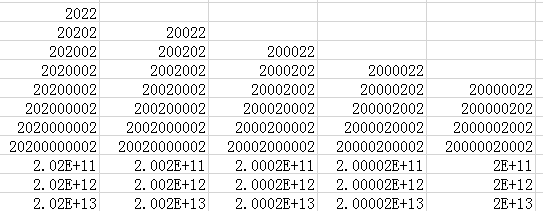但这些数据不全面。 [此贴子已经被作者于2020/2/5 13:36:57编辑过]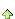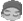2楼 | 信息 | 搜索 | 邮箱 | 主页 | UC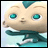加好友发短信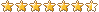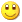Post By：2020/2/5 14:22:00 [只看该作者]

 Dim lst As new List(of Long)Dim i As Long = 0Dim p2 As String = "^[0,2]+\$"Dim r As New System.Text.RegularExpressions.Regex(p2)Do While lst.Count < 2020    If r.IsMatch(i) Then lst.Add(i)    i += 1LoopFor Each s As Long In lst    Output.Show(s)Next3楼 | 信息 | 搜索 | 邮箱 | 主页 | UC加好友发短信Post By：2020/2/5 19:09:00 [只看该作者]

 这段代码我在表属性的DataColChanging事件中执行，直接死机，等很久都没反应！4楼 | 信息 | 搜索 | 邮箱 | 主页 | UC加好友发短信Post By：2020/2/6 10:12:00 [只看该作者]

 首先这种代码不适合在表事件里使用，建议使用按钮处理。其次数据多循环比较久，要等。找到规律改一下，可以提高n倍速度Dim d As Date = Date.NowDim lst As new List(of Long)Dim i As Long = 0Dim p1 As String = "0\$"Dim p2 As String = "^[0,2]+\$"Dim p3 As String = "^2+\$"Dim p4 As String = "0+\$"Dim r As New System.Text.RegularExpressions.Regex(p2)Do While lst.Count < 500    Dim i2 As String= i.Tostring    Dim k As Long = i2.length    If r.IsMatch(i2,p2) Then        lst.Add(i)        If r.IsMatch(i2,p1) Then            i += 2        ElseIf r.IsMatch(i2,p4) Then            Dim s2 As String = System.Text.RegularExpressions.Regex.replace(i2,p4,"")            i=clng(s2 & "2".PadRight(k-s2.Length,"0"))        ElseIf r.IsMatch(i2,p3) Then            i=cint("2".PadRight(k+1,"0"))        Else            i += 18        End If    Else        i += 2    End IfLoopDim t As TimeSpan = Date.Now - dOutput.Show(t.TotalSeconds)For Each s As Long In lst    Output.Show(s)Next5楼 | 信息 | 搜索 | 邮箱 | 主页 | UC加好友发短信Post By：2020/2/6 11:34:00 [只看该作者]

 代码做到按钮里了，执行后一点反应都没有，我想把生成的数据全部显示在表中，因为题目要求还要对前2020个鼠数进行统计，所以D不必设为日期型，生成的数据能否设为长数字型，这样才能进行统计。6楼 | 信息 | 搜索 | 邮箱 | 主页 | UC加好友发短信Post By：2020/2/6 11:52:00 [只看该作者]

 我的代码只是输出到Output.Show里，如果要保存到表请先看懂代码自己改改。d变量是为了测试代码执行的速度的，不需要可以去掉。还是那句话，先看懂代码再说。7楼 | 信息 | 搜索 | 邮箱 | 主页 | UC加好友发短信Post By：2020/2/6 19:44:00 [只看该作者]

 我把你的代码在命令窗口中执行没错，但把500改为2020报错，在按扭上改成这样，执行的结果全是222220022，请教问题出在哪呢？'Dim d As LongDim lst As new List(of Long)Dim i As Long = 0Dim p1 As String = "0\$"Dim p2 As String = "^[0,2]+\$"Dim p3 As String = "^2+\$"Dim p4 As String = "0+\$"Dim r As New System.Text.RegularExpressions.Regex(p2)Do While lst.Count < 2020    Dim i2 As String= i.Tostring    Dim k As Long = i2.length    If r.IsMatch(i2,p2) Then        lst.Add(i)        If r.IsMatch(i2,p1) Then            i += 2        ElseIf r.IsMatch(i2,p4) Then            Dim s2 As String = System.Text.RegularExpressions.Regex.replace(i2,p4,"")            i=clng(s2 & "2".PadRight(k-s2.Length,"0"))        ElseIf r.IsMatch(i2,p3) Then            i=cint("2".PadRight(k+1,"0"))        Else            i += 18        End If    Else        i += 2    End IfLoop'Dim t As TimeSpan = Date.Now - d'Output.Show(t.TotalSeconds)For Each s As Long In lst    'Output.Show(s)For Each dr As DataRow In DataTables("表A").DataRows    dr("鼠数") = sNextNext [此贴子已经被作者于2020/2/6 19:45:41编辑过]8楼 | 信息 | 搜索 | 邮箱 | 主页 | UC加好友发短信Post By：2020/2/7 11:34:00 [只看该作者]

 多看看基础的内容：http://www.foxtable.com/webhelp/topics/1592.htmDim dr As DataRowFor Each s As Long In lst    'Output.Show(s)    dr = DataTables("表A").AddNew    dr("鼠数") = sNext9楼 | 信息 | 搜索 | 邮箱 | 主页 | UC加好友发短信Post By：2020/2/7 11:38:00 [只看该作者]

 我把代码改为这样：'Dim d As LongDim lst As new List(of Long)Dim i As Long = 0Dim p1 As String = "0\$"Dim p2 As String = "^[0,2]+\$"Dim p3 As String = "^2+\$"Dim p4 As String = "0+\$"Dim r As New System.Text.RegularExpressions.Regex(p2)Do While lst.Count < 2020    Dim i2 As String= i.Tostring    Dim k As Long = i2.length    If r.IsMatch(i2,p2) Then        lst.Add(i)        If r.IsMatch(i2,p1) Then            i += 2        ElseIf r.IsMatch(i2,p4) Then            Dim s2 As String = System.Text.RegularExpressions.Regex.replace(i2,p4,"")            i=clng(s2 & "2".PadRight(k-s2.Length,"0"))        ElseIf r.IsMatch(i2,p3) Then            i=cint("2".PadRight(k+1,"0"))        Else            i += 18        End If    Else        i += 2    End IfLoop'Dim t As TimeSpan = Date.Now - d'Output.Show(t.TotalSeconds)For Each s As Long In lst    'Output.Show(s)    For Each dr As DataRow In DataTables("表A").DataRows        For a As Integer = 0 To lst.count-1            dr = dr(a)            dr("鼠数") = dr("鼠数")(a) = s        Next    NextNext报错：此主题相关图片如下：qq图片1.png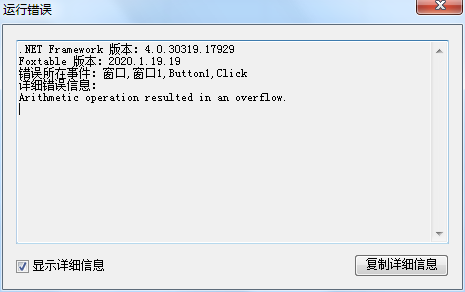10楼 | 信息 | 搜索 | 邮箱 | 主页 | UC加好友发短信Post By：2020/2/7 11:41:00 [只看该作者]

 哦，会不会象你的代码在命令中执行如果改为2020就报错，实际上是没法执行这么大的数据？总数 35 1 2 3 4 下一页